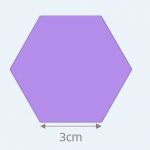Smartick is a fun way to learn math!I want to learn about: Geometry

Learn elementary geometry with Smartick. Exercises, theory, tutorials, and teaching resources for children learning elementary geometry. Here you will find posts about geometry to make learning easier and more fun.

Mar11

Consecutive Interior Angles: What They Are and How to Find Them With Examples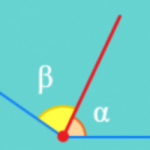In this post, we are going to discover what consecutive interior angles are and how to differentiate them from other types of angles. You will see some examples and even a little quiz. But before we start, let’s review what an angle is. An angle is the portion of a plane between two rays that […]

Jan15

What is an Obtuse Angle?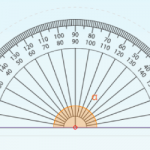In today’s post, we are going to learn about what an obtuse angle is with different examples taken from everyday life and some Smartick exercises. What is an Obtuse Angle? An angle is a space shared by two rays that are joined at the same vertex. We can find different types of angles according to […]

Dec28

Complementary Angles: What Are They?In today’s post, we are going to learn about what complementary angles are and their properties. After, we will look at some examples and complete a few exercises together. Before we begin, we would recommend reviewing the types of angles that exist. What Are Complementary Angles? Two angles are complementary if the sum of the […]

Nov26

Perimeter: What Is It and How to Find It for Any Polygon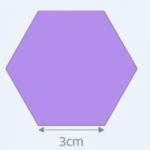Today we are going to learn to calculate perimeters of geometric figures. But first, let’s start with the definition. What is a Perimeter? A perimeter is what we call the length of the outline of a geometric figure. Therefore, the perimeter is a measurement of length, so it will come in centimeters, inches, and feet, […]

Oct30

What is a Straight Angle and ExamplesIn today’s post, we will learn what a straight angle is, how many degrees it has, and its properties. We will also practice with some examples. What is a Straight Angle? An angle is a portion of the plane between two rays that are joined by a vertex. A straight angle is the shared space […]

Apr13

Elementary Geometry – Open LinesIn this post, we are going to discover what an open line is and how it is different from a closed line. We will also see different types of open lines. Before we begin, take a look at this post about straight and curved lines to refresh your memory. Open Lines We know that a […]

Mar23

Identifying Flat Symmetrical Figures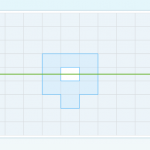In today’s post, I am going to present a series of exercises to practice how to identify flat symmetrical figures. To begin, I recommend that if you don’t remember symmetry or the characteristics that make a figure symmetrical very well, take a look at this previous post. You’ll be able to review all of the […]

Jan06

How to Calculate a Perimeter: Part 2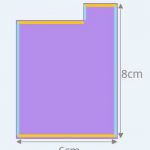In an earlier post, we learned how to calculate the perimeter of any polygon and formulas for regular polygons and rectangles. Today, we are going to learn how to calculate the perimeter of other types of polygons: rhombuses, isosceles triangles, isosceles trapezoids, and stepped polygons. NOTE TO OUR READERS IN THE U.K. – This post […]

Oct28

Calculating the Area of Polygons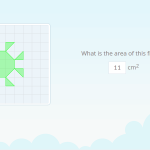In today’s post, I am going to show you some exercises we have recently incorporated into Smartick for calculating the area of polygons. For some time we have had exercises to practice the area of figures with small squares. The way to calculate the area of these figures is very simple because all you have […]

Oct14

What Is an Angle? How Is It Made?In today’s post we are going to answer the question, what is an angle? To begin, it would be a good idea for you to review the following link where we have explained the concept of ”straight lines.” Types of Lines Now that you remember straight lines, let’s begin! In order to understand what an […]

Aug19

Do You Know What a Right Angle Is?

You probably already have some idea about what we call a right angle. But, I still would recommend taking a look at some other posts on our blog before continuing, for example, you can review this post about the different types of angles. Are you ready? Well, let’s expand your knowledge about right angles. Imagine […]